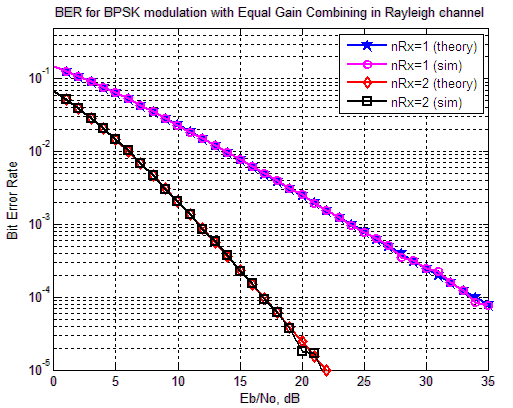- DSP log - http://www.dsplog.com -

Equal Gain Combining (EGC)

Posted By Krishna Sankar On September 19, 2008 @ 10:53 pm In MIMO | 42 Comments

This is the second post in the series discussing receiver diversity in a wireless link. Receiver diversity is a form of space diversity, where there are multiple antennas at the receiver. The presence of receiver diversity poses an interesting problem – how do we use ‘effectively‘ the information from all the antennas to demodulate the data. In the previous post, we discussed selection diversity . In this post, we will discuss equal gain combining (EGC). For the discussion, we will assume that the channel is a flat fading Rayleigh multipath channel  and the modulation is BPSK.

## Background

We use the same constraints as defined in the Selection Diversity  post. Let me repeat the same.

1. We have N receive antennas and one transmit antenna.

2. The channel is flat fading – In simple terms, it means that the multipath channel has only one tap. So, the convolution operation reduces to a simple multiplication. For a more rigorous discussion on flat fading and frequency selective fading, may I urge you to review Chapter 15.3 Signal Time-Spreading from [DIGITAL COMMUNICATIONS: SKLAR]3. The channel experienced by each receive antenna is randomly varying in time. For the receive antenna, each transmitted symbol gets multiplied by a randomly varying complex number . As the channel under consideration is a Rayleigh channel, the real and imaginary parts of are Gaussian distributed having mean and variance .

4. The channel experience by each receive antenna is independent from the channel experienced by other receive antennas.

5. On each receive antenna, the noise has the Gaussian probability density function with

with and .

The noise on each receive antenna is independent from the noise on the other receive antennas.

6. At each receive antenna, the channel is known at the receiver.

7. In the presence of channel , the instantaneous bit energy to noise ratio at receive antenna is . For notational convenience, let us define,

.

From the discussion on chi-square random variable , we know that, if is a Rayleigh distributed random variable, then is a chi-squared random variable with two degrees of freedom. The pdf of is
.

## Equal Gain Combining (EGC)

On the receive antenna, equalization is performed at the receiver by dividing the received symbol by the apriori known phase of . The channel is represented in polar form as . The decoded symbol is the sum of the phase compensated channel from all the receive antennas.

where
is the additive noise scaled by the phase of the channel coefficient.

For demodulation, we use the classical definition i.e.

and

Note:

For PSK modulation schemes, the equalization by the phase of the channel coefficients suffice. However, for QAM case, we need to compensate for the amplitude also when equalizing. We are NOT discussing QAM case in this post.

## Effective Eb/N0 with Equal Gain Combining

The equations listed below obtained from the article Receive diversity – Notes by Prof. Raviraj Adve .

In the presence of channel , the instantaneous bit energy to noise ratio at receive antenna is . For notational convenience, let us define,

.

The effective Eb/N0 with equal gain combining is the channel power accumulated over all receive chains, i.e.

.

The first term is chi-square random variable with degrees of freedom having mean value of . Hence the first term reduces to,

.

The second term is a product of two Rayleigh random variables. The mean of Rayleigh random variable with variance is . Hence the second term is,

.

Simplifying, the effective Eb/N0 with equal gain combining is,

.Figure : Gain in Eb/N0 with equal gain combining

## Bit Error Rate with Equal Gain Combining

The IEEE paper [ZHANG97] discuss the BER computation with Equal Gain Combining. Since the proof is tedious (and I did not understand) I am just noting the final results.

With two receive antennas, the BER with equal gain combining is,

.

## Matlab model for simulating BER with Equal Gain Combining

The Matlab/Octave script performs the following

(a) Generate random binary sequence of +1′s and -1′s.

(b) Multiply the symbols with the channel and then add white Gaussian noise.

(c) At the receiver, for each receive path, equalize by compensating with the known channel phase

(d) Accumulate the equalized symbols from all the receive paths

(d) Perform hard decision decoding and count the bit errors

(e) Repeat for multiple values of and plot the simulation and theoretical results.Figure: BER plot Receive Diversity with Equal Gain Combining

Can observe that the simulation results are in good agreement with the theoretical results.## Reference

[ZHANG97] Probability of error for equal-gain combiners over Rayleigh channels: some closed-form solutions  Zhang, Q.T. Communications, IEEE Transactions on Volume 45, Issue 3, Date: Mar 1997, Pages: 270 – 273

URL to article: http://www.dsplog.com/2008/09/19/equal-gain-combining/

URLs in this post:

 Rayleigh multipath channel: http://www.dsplog.com/2008/07/14/rayleigh-multipath-channel/

 chi-square random variable: http://www.dsplog.com/2008/07/28/chi-square-random-variable/#twodegree